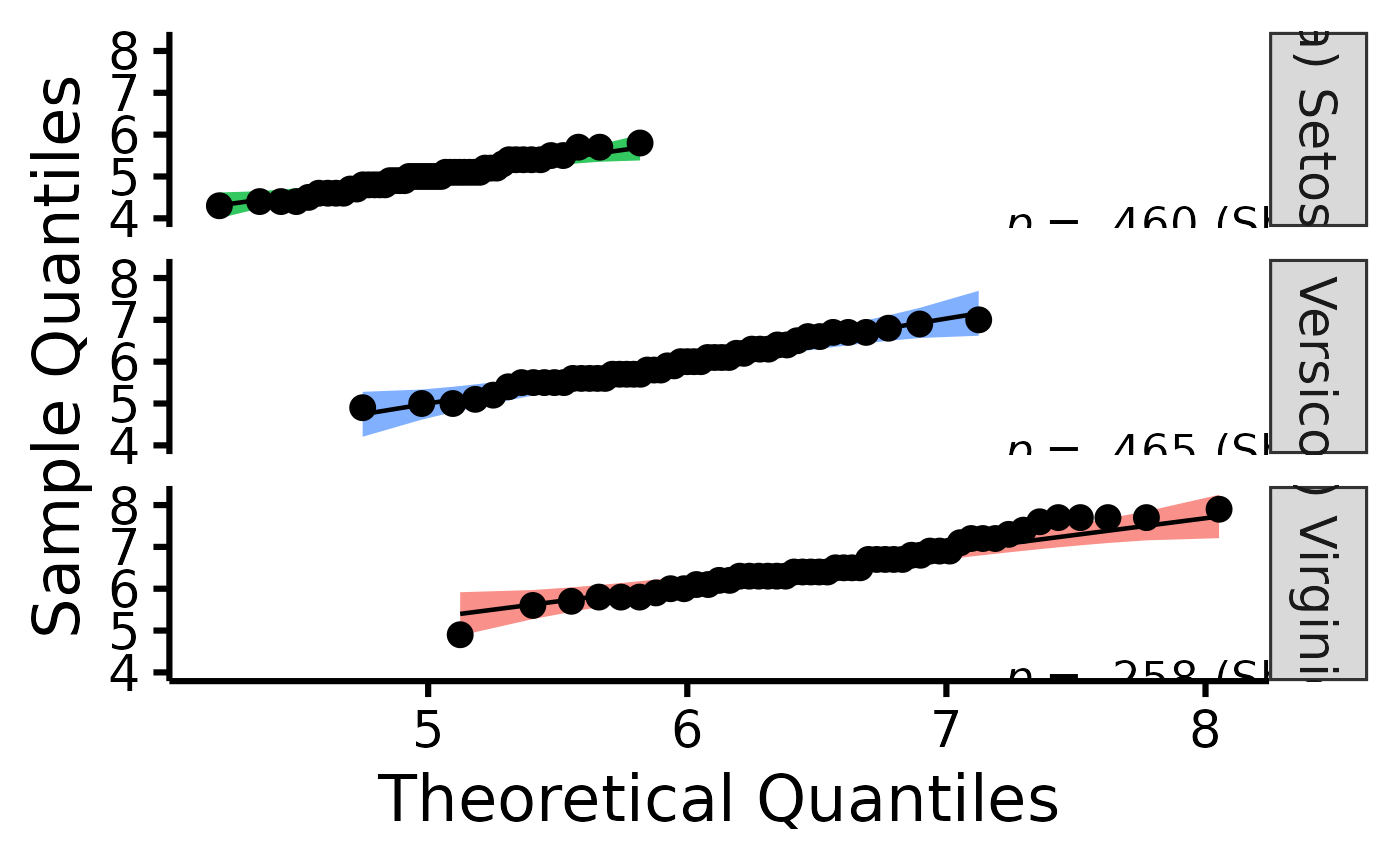Easily make nice per-group QQ plots through a wrapper around the ggplot2 and qqplotr packages.

## Usage

nice_qq(
data,
variable,
group = NULL,
colours,
groups.labels = NULL,
grid = TRUE,
shapiro = FALSE,
title = variable
)

## Arguments

data

The data frame.

variable

The dependent variable to be plotted.

group

The group by which to plot the variable.

colours

Desired colours for the plot, if desired.

groups.labels

How to label the groups.

grid

Logical, whether to keep the default background grid or not. APA style suggests not using a grid in the background, though in this case some may find it useful to more easily estimate the slopes of the different groups.

shapiro

Logical, whether to include the p-value from the Shapiro-Wilk test on the plot.

title

An optional title, if desired.

## Value

A qq plot of class ggplot, by group (if provided), along a reference interpretation helper, the 95% confidence band.

Other functions useful in assumption testing: nice_assumptions, nice_density, nice_normality, nice_var, nice_varplot. Tutorial: https://rempsyc.remi-theriault.com/articles/assumptions

## Examples

# Make the basic plot
nice_qq(
data = iris,
variable = "Sepal.Length",
group = "Species"
)# Further customization
nice_qq(
data = iris,
variable = "Sepal.Length",
group = "Species",
colours = c("#00BA38", "#619CFF", "#F8766D"),
groups.labels = c("(a) Setosa", "(b) Versicolor", "(c) Virginica"),
grid = FALSE,
shapiro = TRUE,
title = NULL
)